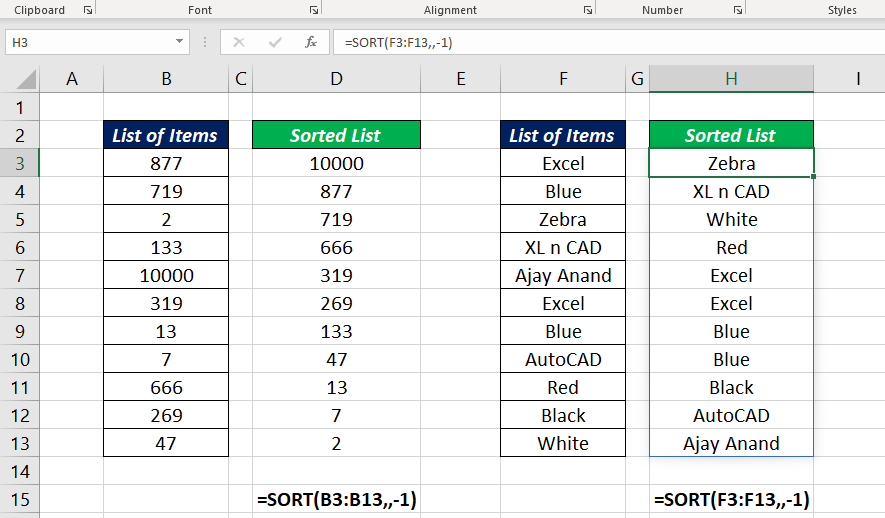# SORT FunctionThe Excel SORT function is a DYNAMIC ARRAY FUNCTION used to sort the contents of an array or range of cells. Single to Multi-dimensional arrays/ranges can be sorted by one or more columns using the SORT function.

SORT function is a Dynamic Array function, which means the result returned by SORT function is a dynamic Array.

### Function Type

Lookup and reference

### Purpose

Sort the values of an array or range

### Return value

Sorted array of values

### Syntax

`=SORT (array, [sort_index], [sort_order], [by_col]) `

### Arguments

array – array or range to sort

sort_index – [optional] column index for sorting. Default value is 1.

sort_order – [optional] 1 for Ascending order and -1 for Descending order. The default order is ascending

by_col – [optional] FALSE to sort by row and TRUE to sort by column.

### Examples

#### Example 1

To sort the list of items in the range B3:B13 use the following formula

`=SORT(B3:B13)`

The items in the list sorted in Alphabetical order.

#### Example 2

Now, an example of sorting numbers using SORT function. To sort the list of items (Ascending order) in the range B3:B13 use the following formula

`=SORT(B3:B13)`

The numbers in the list sorted in Ascending order.

#### Example 3

To change the order of sorting i.e. to sort the items in Descending order or Reverse Alphabetical order, use -1 in the place of the third argument, sort_order

` =SORT(B3:B13,,-1)`

The above formula will sort the numbers in the range B3:B13, in descending order

`=SORT(F3:F13,,-1)`

The formula above will sort the words in the range F3:F13, in reverse Alphabetical order

#### Example 4

To sort the data in a horizontal data range, use TRUE or 1 in the place of the fourth argument by_col

To sort the values in the horizontal data range C2:I2, use the following formula

`=SORT(C2:I2,,,TRUE)`

Two more examples in which the SORT function is used to sort records containing multiple columns.

#### Example 5

The following formula will sort the data in the range B3:B17

`=SORT(B3:E17)`

Here the data is sorted according to values in column 1

#### Example 6

To sort the entire data according to the values in the column for SalesRep, I mean the third column, use the value 3 in the place of the second argument sort_index

`=SORT(B3:E17,3)`

The above formula will sort the data in the order of values in the third column

### Note

The Excel SORT function sort values in a range and the result is a dynamic array of values. This array values will ‘spill’ onto the worksheet into a range that automatically updates when new values are added to or removed from the source range. So, be careful while using SORT function. If this spill range contains any data, the function will return a #SPILL! error.